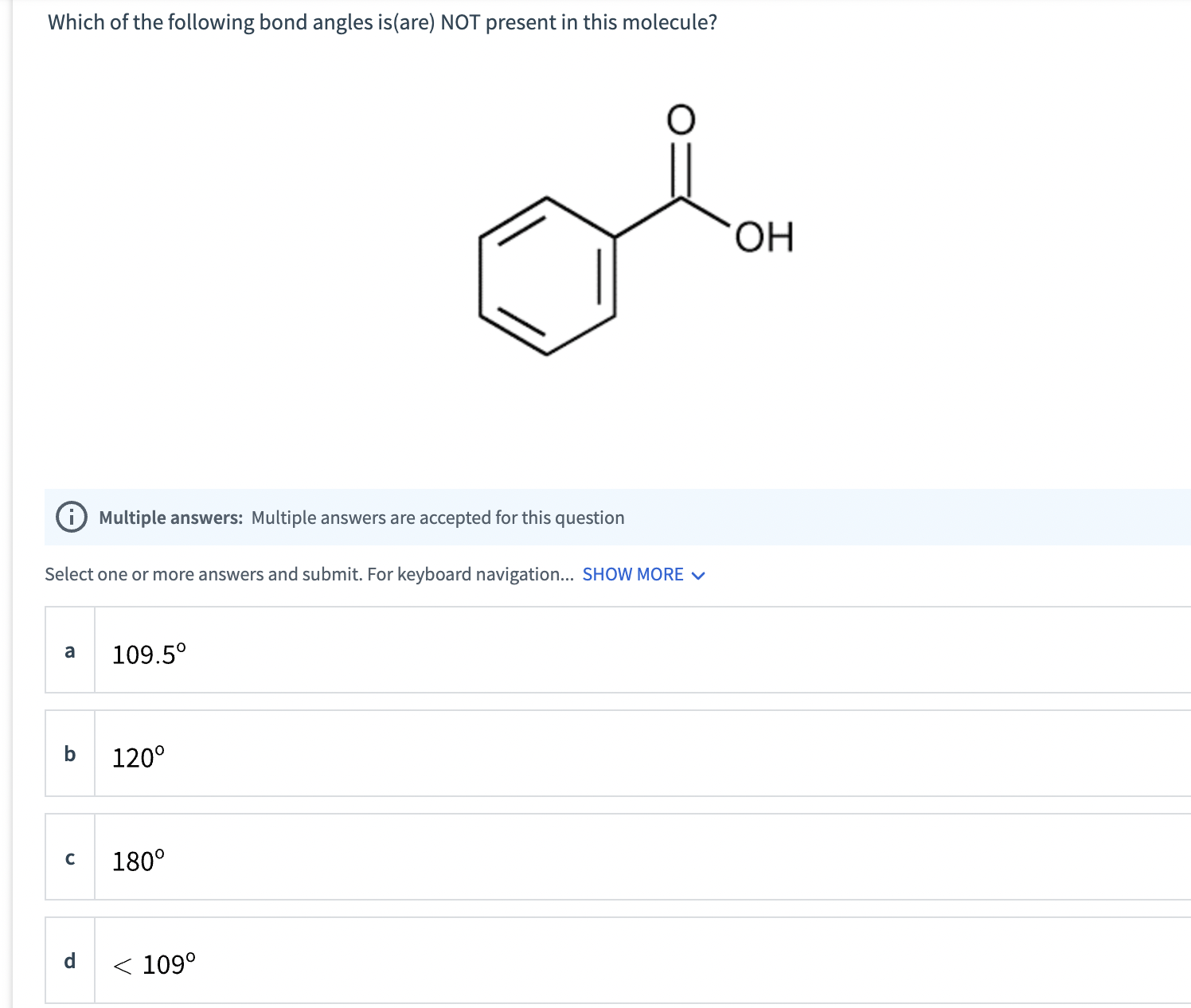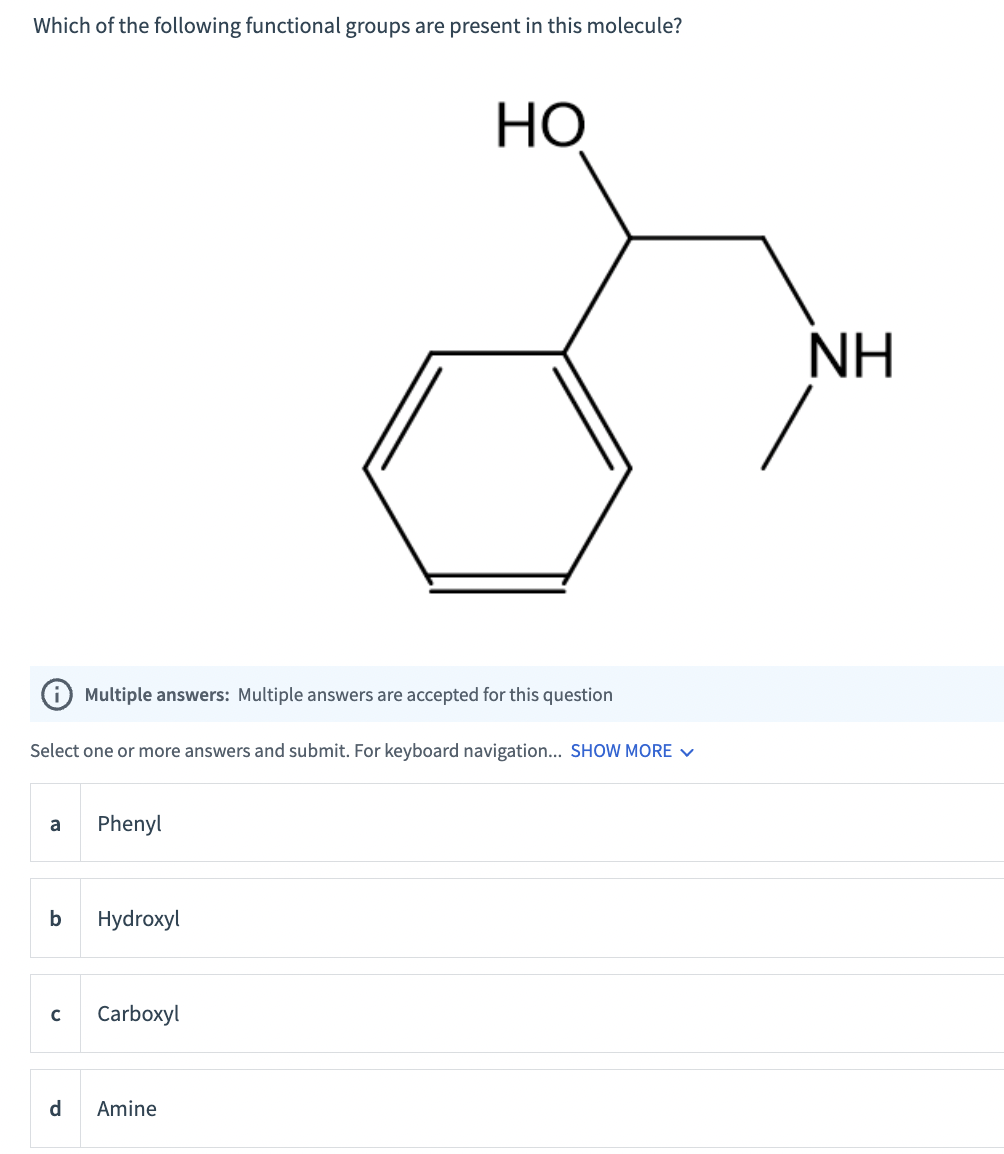# Question Which of the following bond angles is(are) NOT present in this molecule? (i) Multiple answers: Multiple answers are accepted for this question Select one or more answers and submit. For keyboard navigation... SHOW MORE $$\checkmark$$ a $$109.5^{\circ}$$ b $$120^{\circ}$$ c $$180^{\circ}$$ d $$<109^{\circ}$$ Which of the following functional groups Which of the following bond angles is(are) NOT present in this molecule? (i) Multiple answers: Multiple answers are accepted for this question Select one or more answers and submit. For keyboard navigation... SHOW MORE $$\checkmark$$ a $$109.5^{\circ}$$ b $$120^{\circ}$$ c $$180^{\circ}$$ d $$<109^{\circ}$$ Which of the following functional groups are present in this molecule? (i) Multiple answers: Multiple answers are accepted for this question Select one or more answers and submit. For keyboard navigation... SHOW MORE $$\vee$$ a Phenyl b Hydroxyl c Carboxyl d Amine1.2.Transcribed Image Text: Which of the following bond angles is(are) NOT present in this molecule? (i) Multiple answers: Multiple answers are accepted for this question Select one or more answers and submit. For keyboard navigation... SHOW MORE $$\checkmark$$ a $$109.5^{\circ}$$ b $$120^{\circ}$$ c $$180^{\circ}$$ d $$<109^{\circ}$$ Which of the following functional groups are present in this molecule? (i) Multiple answers: Multiple answers are accepted for this question Select one or more answers and submit. For keyboard navigation... SHOW MORE $$\vee$$ a Phenyl b Hydroxyl c Carboxyl d Amine
More
Transcribed Image Text: Which of the following bond angles is(are) NOT present in this molecule? (i) Multiple answers: Multiple answers are accepted for this question Select one or more answers and submit. For keyboard navigation... SHOW MORE $$\checkmark$$ a $$109.5^{\circ}$$ b $$120^{\circ}$$ c $$180^{\circ}$$ d $$<109^{\circ}$$ Which of the following functional groups are present in this molecule? (i) Multiple answers: Multiple answers are accepted for this question Select one or more answers and submit. For keyboard navigation... SHOW MORE $$\vee$$ a Phenyl b Hydroxyl c Carboxyl d Amine